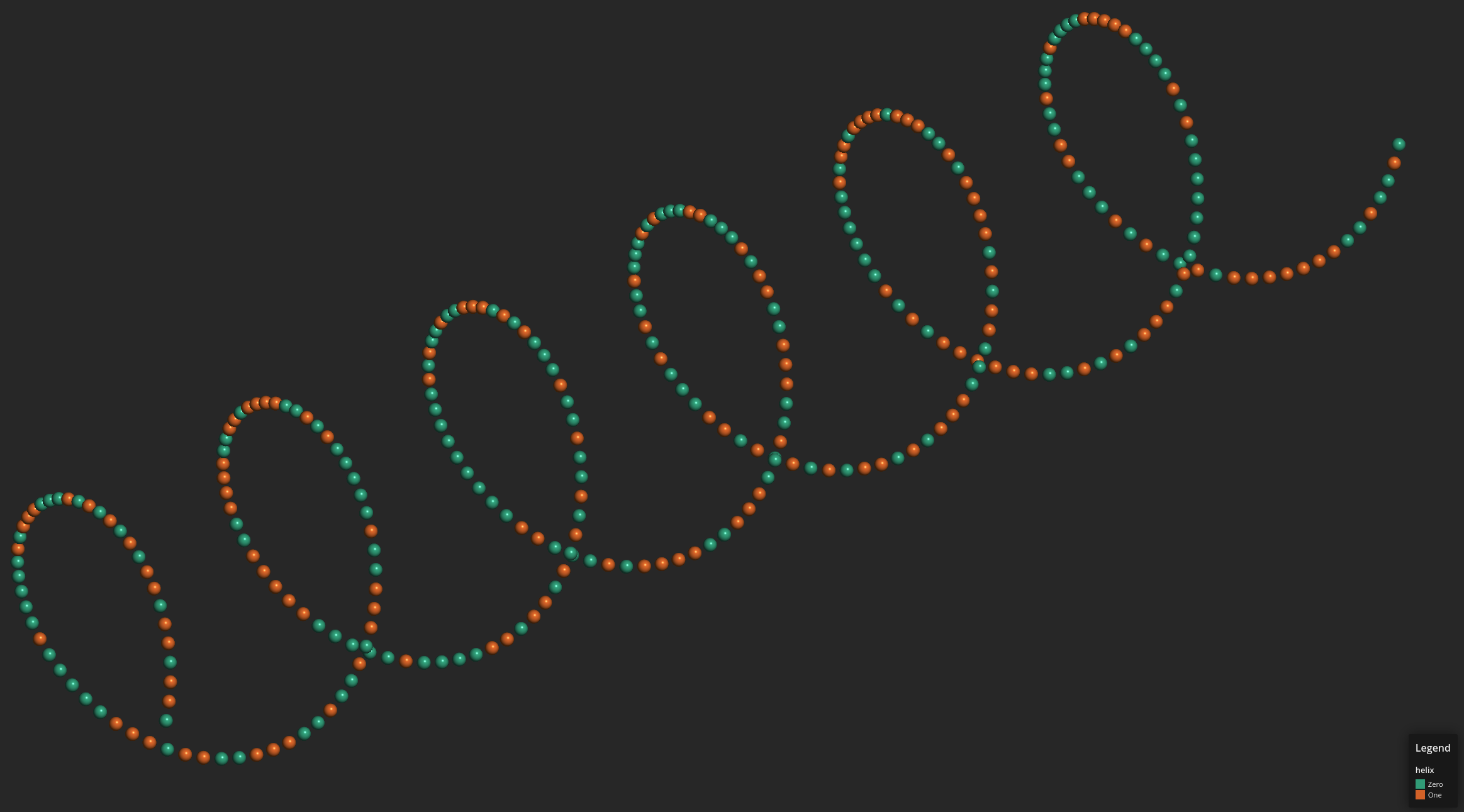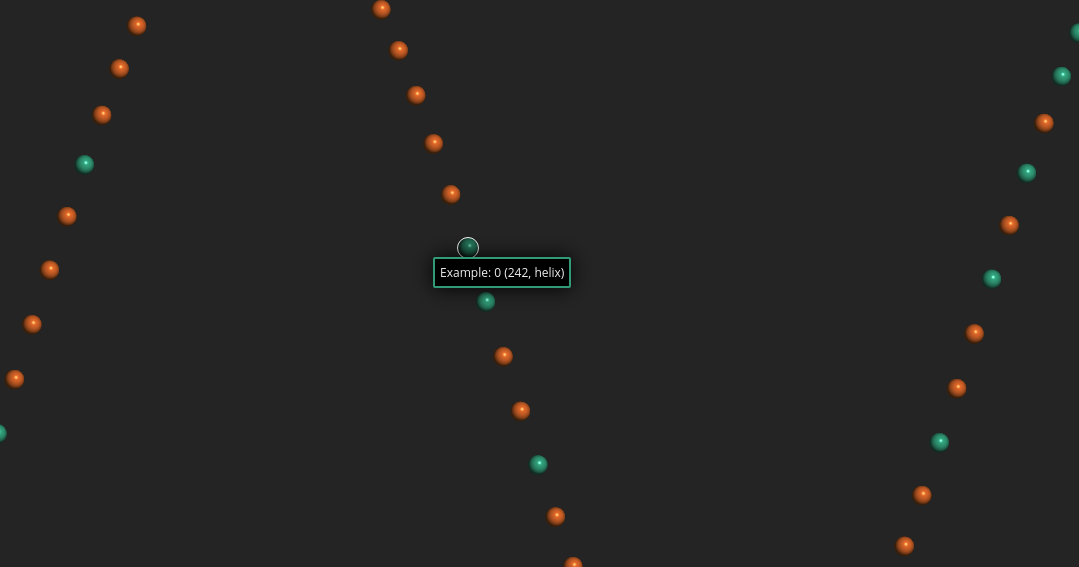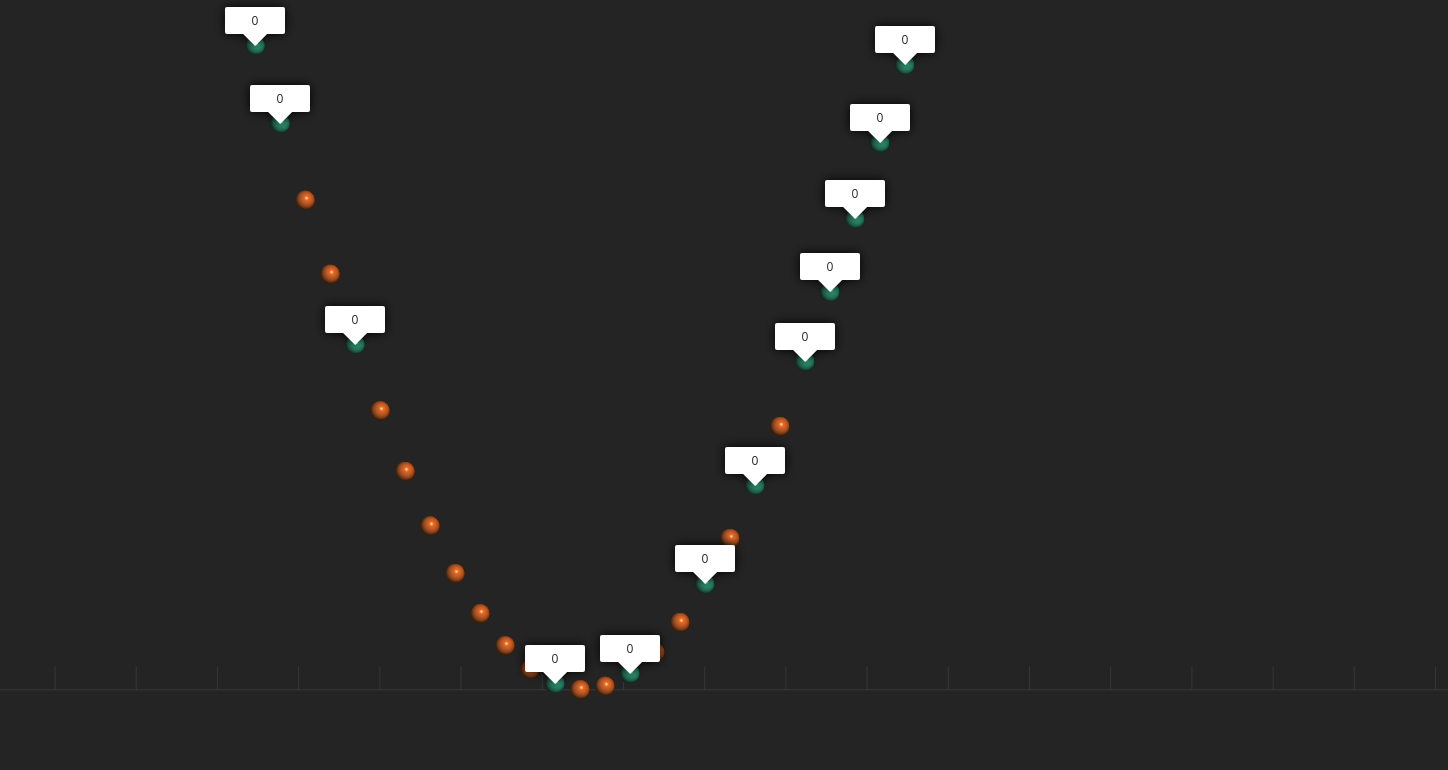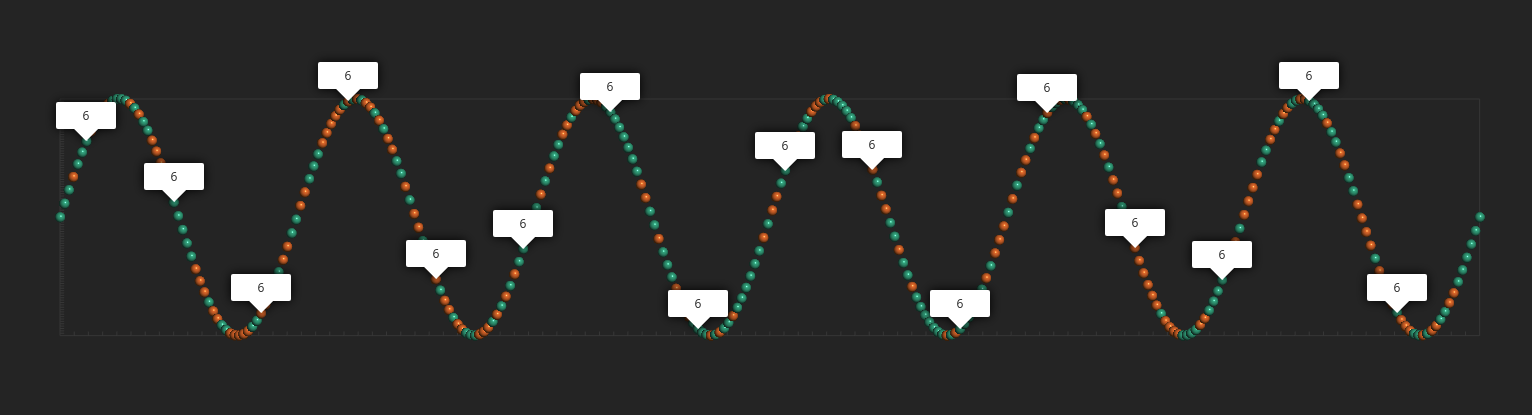# reymond-group / faerun-python

A python module for generating interactive views of chemical spaces.
HTML Python Jupyter NotebookFetching latest commit…
Cannot retrieve the latest commit at this time.

## Files

Type Name Latest commit message Commit time
Failed to load latest commit information..vscodedocexamplesfaerunnotebook Sep 17, 2019.gitignoreCHANGESLICENSEMANIFEST.inREADME.mdrequirements.txtsetup.py

# Faerun (Python)

Faerun (Python) is based on the Lore.js 3D WebGL engine for interactive big data rendering and the FUn project. It facilitates the creation of interactive (2D and 3D) HTML plots of chemical data (or chemical spaces). Molecular structures are rendered using SmilesDrawer.## Installation

Faerun can be installed using pip.

`pip install faerun`

In order to use it in a script, the class `Faerun` has to be imported from the package.

`from faerun import Faerun`

That’s it for the installation.

## Getting Started

### Create a Plot Document

In order to plot, a plot document has to be created. To do so, create an instance of the class `Faerun`

```from faerun import Faerun

f = Faerun(title='faerun-example', clear_color='#222222', coords=False, view='free')```

Here, we set the `title` of the plot document. This will be used as the title of the HTML document. The clear color of the canvas, which is the background color of the plot, is set to `'#222222'` (which is the hex-code for a dark gray). The drawing of coordinate axes is disabled by setting `coords=False` and since we want to draw 3D data, the argument `view` is set to `'free'` to enable the user to pan and rotate the plot.

## Preparing Data for Plotting

The next step is to prepare the data which is to be plotted. In the tutorial, we will just generate some nice looking data using `numpy`.

```import numpy as np

x = np.linspace(0, 12.0, 326)
y = np.sin(np.pi * x)
z = np.cos(np.pi * x)
c = np.random.randint(0, 6, len(x))```

This data can then be wrapped in a `dict`. In addition, `DataFrame` from the `pandas` package are also supported by faerun. In the example, the same values are used for the colors `c` and labels `labels`.

`data = {'x': x, 'y': y, 'z': z, 'c': c, 'labels': c}`

Given the `Faerun` instance and the data, a scatter plot can be created using the method `add_scatter`.

```f.add_scatter('helix', data, shader='sphere', colormap='Dark2', point_scale=5.0,
categorical=True, has_legend=True, legend_labels=[(0, 'Zero'), (1, 'One')])```

The data is added as a scatter layer named helix. The chose shader will render the data points as spheres (with diffuse and specular lighting) of size 5.0 with colors from the `matplotlib` colormap `'Dark2'`. As the `c` is categorical, the parameter `categorical` is set to `True`, otherwise `matplotlib` will mess up the values.

Finally, `has_legend=True` adds the scatter layer to the legend and `legend_labels` is a `list` of `tuple`, associating values with a label.

### Saving to HTML

The faerun document can the be plotted to an HTML document with an accompanying JavaScript data file.

`f.plot('helix')`

This saves the plot as `helix.html` and `helix.js`. The files can be opened locally or hosted on any web server.### Saving to Faerun Data File

The faerun document can also be exported to a faerun data file, which in turn can then be hosted using the `web` module.

```import pickle

with open('helix.faerun', 'wb+') as handle:
pickle.dump(f.create_python_data(), handle, protocol=pickle.HIGHEST_PROTOCOL)```

### Complete Example

```import pickle
import numpy as np
from faerun import Faerun

def main():
f = Faerun(title='faerun-example', clear_color='#222222', coords=False, view='free')

x = np.linspace(0, 12.0, 326)
y = np.sin(np.pi * x)
z = np.cos(np.pi * x)
c = np.random.randint(0, 2, len(x))

data = {'x': x, 'y': y, 'z': z, 'c': c, 'labels': c}

categorical=True, has_legend=True, legend_labels=[(0, 'Zero'), (1, 'One')])

f.plot('helix')

with open('helix.faerun', 'wb+') as handle:
pickle.dump(f.create_python_data(), handle, protocol=pickle.HIGHEST_PROTOCOL)

if __name__ == '__main__':
main()```

## Hosted

### Introduction

While small plots can easily be created and stored in a single HTML and JavaScript file, which are then loaded completely in the browsers memory, this is not possible for larger data sets due to browser limitations. In order to solve this problem, Faerun includes a small HTTP server (based on cherrypy) to serve the data to the browser.

### Creating Faerun Data Files

As shown in Getting Started, Faerun can save data as `.faerun` data files using `pickle`.

```with open('helix.faerun', 'wb+') as handle:
pickle.dump(f.create_python_data(), handle, protocol=pickle.HIGHEST_PROTOCOL)```

### Starting a Faerun Web Server

```from faerun import host

host('helix.faerun', label_type='default',
theme='dark')```

### Formatting Labels

Labels can be formatted by defining a custom `label_formatter`. If no `label_formatter` is provided to the `host` function, the default is used:

`label_formatter = lambda label, index, name: label.split('__')`

This default splits the label value on `'__'` to store different labels and enable search on different values the the displayed labels. See “Searching” for details. Defining a custom label formatter is straight forward. As an example, let’s prefix each label with a string and add their index and layer name:

```def custom_label_formatter(label, index, name):
return f'Example: {label} ({index}, {name})'

host('helix.faerun', label_type='default',
theme='dark', label_formatter=custom_label_formatter)```

This function is then called whenever a label is requested from the server. In addition to the argument `label`, the arguments `index` and `name` can be used to further customize the displayed label and represent the integer index of the data point and the data layer they belong to (e.g. the name defined with `add_scatter`).Faerun allows to link the data to an arbitrary URL which can be visited upon double-clicking a data point. In order to do this, a `link_formatter` has to be provided. This works similar to customizing the label.

```def custom_link_formatter(label, index, name):

host('helix.faerun', label_type='default',

### Searching

The hosted version of a Faerun visualization also allows for searching. As a default, the search searches for exact matches in labels (substring or regex searches are not possible at this time).However, the search can be customized. As described in “Formatting Labels”, additional label values can be added by separating them using `'__'`.

```c = np.random.randint(0, 2, len(x))
labels = [''] * len(c)

for i, e in enumerate(c):
labels[i] = str(e) + '__' + str(i % 20)

data = {'x': x, 'y': y, 'z': z, 'c': c, 'labels': labels}```

The above examples adds an additional label value and as default, the second label value is then used by the search.If there are additional label values, the search index can be set using the `search_index` argument.

As the visualization is ready to be deployed to a publicly accessible web server, it might be of interest to add a documentation. The `host` method supports the argument `info` that accepts a (markdown formatted) string. This information is the desplayed on the generated web page.

```info = ('#Welcome to Fearun',
'This is a small Faerun example.'
'',
'Yay markdown! This means that you can easily:',
'- Build tables',
'- ...'
)

host('helix.faerun', label_type='default', theme='dark',
info='\n'.join(info))```

An info button is then shown next to the screenshot button, which upon click opens a window containing the info.

### Complete Example

```import pickle
import numpy as np
from faerun import Faerun, host

def main():
f = Faerun(title='faerun-example', clear_color='#222222', coords=False, view='free')

x = np.linspace(0, 12.0, 326)
y = np.sin(np.pi * x)
z = np.cos(np.pi * x)
c = np.random.randint(0, 2, len(x))

labels = [''] * len(c)

for i, e in enumerate(c):
labels[i] = str(e) + '__' + str(i % 20)

data = {'x': x, 'y': y, 'z': z, 'c': c, 'labels': labels}

categorical=True, has_legend=True, legend_labels=[(0, 'Zero'), (1, 'One')])

f.plot('helix')

with open('helix.faerun', 'wb+') as handle:
pickle.dump(f.create_python_data(), handle, protocol=pickle.HIGHEST_PROTOCOL)

def custom_label_formatter(label, index, name):
return f'Example: {label} ({index}, {name})'

info = ('#Welcome to Fearun',
'This is a small Faerun example.'
'',
'Yay markdown! This means that you can easily:',
'- Build tables',
'- ...'
)

host('helix.faerun', label_type='default', theme='dark',
info='\n'.join(info))

if __name__ == '__main__':
main()```

## Examples

Furthermore, the documentation of tmap provides a wide range of examples of Faerun in use. See here.

## Documentation

### Faerun

```class faerun.Faerun(title: str = '', clear_color: str = '#111111', coords: bool = True,
coords_color: str = '#888888', coords_box: bool = False,
coords_ticks: bool = True, coords_grid: bool = False,
coords_tick_count: int = 10, coords_tick_length: float = 2.0,
coords_offset: float = 5.0, x_title: str = '', y_title: str = '',
show_legend: bool = True, legend_title: str = 'Legend',
legend_orientation: str = 'vertical', legend_number_format: str = '{:.2f}',
view: str = 'free', scale: float = 750.0, alpha_blending: bool = False,
style: Dict[str, Dict[str, Any]] = {})```

Creates a faerun object which is an empty plotting surface where layers such as scatter plots can be added.

Constructor for Faerun.

• Keyword Arguments

• title (`str`, optional) – The plot title

• clear_color (`str`, optional) – The background color of the plot

• coords (`bool`, optional) – Show the coordinate axes in the plot

• coords_color (`str`, optional) – The color of the coordinate axes

• coords_box (`bool`, optional) – Show a box around the coordinate axes

• coords_tick (`bool`, optional) – Show ticks on coordinate axes

• coords_grid (`bool`, optional) – Extend ticks to create a grid

• coords_tick_count (`int`, optional) – The number of ticks to display per axis

• coords_tick_length (`float`, optional) – The length of the coordinate ticks

• coords_offset (`float`, optional) – An offset added to the coordinate axes

• x_title (`str`, optional) – The title of the x-axis

• y_title (`str`, optional) – The title of the y-axis

• show_legend (`bool`, optional) – Whether or not to show the legend

• legend_title (`str`, optional) – The legend title

• legend_orientation (`str`, optional) – The orientation of the legend (‘vertical’ or ‘horizontal’)

• legend_number_format (`str`, optional) – A format string applied to the numbers displayed in the legend

• view (`str`, optional) – The view (front, back, top, bottom, left, right, free)

• scale (`float`, optional) – To what size to scale the coordinates (which are normalized)

• alpha_blending (`bool`, optional) – Whether to activate alpha blending (required for smoothCircle shader)

• style (`Dict[str, Dict[str, Any]]`, optional) – The css styles to apply to the HTML elements

```__init__(title: str = '', clear_color: str = '#111111', coords: bool = True,
coords_color: str = '#888888', coords_box: bool = False, coords_ticks: bool = True,
coords_grid: bool = False, coords_tick_count: int = 10, coords_tick_length: float = 2.0,
coords_offset: float = 5.0, x_title: str = '', y_title: str = '', show_legend: bool = True,
legend_title: str = 'Legend', legend_orientation: str = 'vertical',
legend_number_format: str = '{:.2f}', view: str = 'free', scale: float = 750.0,
alpha_blending: bool = False, style: Dict[str, Dict[str, Any]] = {})```

Constructor for Faerun.

• Keyword Arguments

• title (`str`, optional) – The plot title

• clear_color (`str`, optional) – The background color of the plot

• coords (`bool`, optional) – Show the coordinate axes in the plot

• coords_color (`str`, optional) – The color of the coordinate axes

• coords_box (`bool`, optional) – Show a box around the coordinate axes

• coords_tick (`bool`, optional) – Show ticks on coordinate axes

• coords_grid (`bool`, optional) – Extend ticks to create a grid

• coords_tick_count (`int`, optional) – The number of ticks to display per axis

• coords_tick_length (`float`, optional) – The length of the coordinate ticks

• coords_offset (`float`, optional) – An offset added to the coordinate axes

• x_title (`str`, optional) – The title of the x-axis

• y_title (`str`, optional) – The title of the y-axis

• show_legend (`bool`, optional) – Whether or not to show the legend

• legend_title (`str`, optional) – The legend title

• legend_orientation (`str`, optional) – The orientation of the legend (‘vertical’ or ‘horizontal’)

• legend_number_format (`str`, optional) – A format string applied to the numbers displayed in the legend

• view (`str`, optional) – The view (front, back, top, bottom, left, right, free)

• scale (`float`, optional) – To what size to scale the coordinates (which are normalized)

• alpha_blending (`bool`, optional) – Whether to activate alpha blending (required for smoothCircle shader)

• style (`Dict[str, Dict[str, Any]]`, optional) – The css styles to apply to the HTML elements

```add_scatter(name: str, data: Union[dict, pandas.core.frame.DataFrame],
mapping: dict = {'c': 'c', 'cs': 'cs', 'labels': 'labels', 's': 's', 'x': 'x', 'y': 'y', 'z': 'z'},
colormap: Union[str, matplotlib.colors.Colormap] = 'plasma', shader: str = 'sphere',
point_scale: float = 1.0, max_point_size: float = 100.0, fog_intensity: float = 0.0,
saturation_limit: float = 0.2, categorical: bool = False, interactive: bool = True,
has_legend: bool = False, legend_title: str = None, legend_labels: dict = None,
min_legend_label: Union[str, float] = None, max_legend_label: Union[str, float] = None)```

Add a scatter layer to the plot.

• Parameters

• name (`str`) – The name of the layer

• data (`dict` or `DataFrame`) – A Python dict or Pandas DataFrame containing the data

• Keyword Arguments

• mapping (`dict`, optional) – The keys which contain the data in the input dict or the column names in the pandas `DataFrame`

• colormap (`str` or `Colormap`, optional) – The name of the colormap (can also be a matplotlib Colormap object)

• shader (`str`, optional) – The name of the shader to use for the data point visualization

• point_scale (`float`, optional) – The relative size of the data points

• max_point_size (`int`, optional) – The maximum size of the data points when zooming in

• fog_intensity (`float`, optional) – The intensity of the distance fog

• saturation_limit (`float`, optional) – The minimum saturation to avoid “gray soup”

• categorical (`bool`, optional) – Whether this scatter layer is categorical

• interactive (`bool`, optional) – Whether this scatter layer is interactive

• has_legend (`bool`, optional) – Whether or not to draw a legend

• legend_title (`str`, optional) – The title of the legend

• legend_labels (`dict`, optional) – A dict mapping values to legend labels

• min_legend_label (`Union[str, float]`, option) – The label used for the miminum value in a ranged (non-categorical) legend

• max_legend_label (`Union[str, float]`, option) – The label used for the maximum value in a ranged (non-categorical) legend

```add_tree(name: str, data: Union[dict, pandas.core.frame.DataFrame],
mapping: dict = {'c': 'c', 'from': 'from', 'to': 'to', 'x': 'x', 'y': 'y', 'z': 'z'},
color: str = '#666666', colormap: Union[str, matplotlib.colors.Colormap] = 'plasma',
fog_intensity: float = 0.0, point_helper: str = None)```

Add a tree layer to the plot.

• Parameters

• name (`str`) – The name of the layer

• data (`dict` or `DataFrame`) – A Python dict or Pandas DataFrame containing the data

• Keyword Arguments

• mapping (`dict`, optional) – The keys which contain the data in the input dict or DataFrame

• color (`str`, optional) – The default color of the tree

• colormap (`str` or `Colormap`, optional) – The name of the colormap (can also be a matplotlib Colormap object)

• fog_intensity (`float`, optional) – The intensity of the distance fog

• point_helper (`str`, optional) – The name of the scatter layer to associate with this tree layer (the source of the coordinates)

`create_data()`

Returns a JavaScript string defining a JavaScript object containing the data.

• Returns

JavaScript code defining an object containing the data

• Return type

`str`

`create_python_data()`

Returns a Python dict containing the data

• Returns

The data defined in this Faerun instance

• Return type

`dict`

`static discrete_cmap(n_colors: int, base_cmap: str)`

Create an N-bin discrete colormap from the specified input map.

• Parameters

n_colors (`int`) – The number of discrete colors to generate

• Keyword Arguments

base_cmap (`str`) – The colormap on which to base the discrete map

• Returns

The discrete colormap

• Return type

`Colormap`

`get_min_max()`

Get the minimum an maximum coordinates from this plotter instance

• Returns

The minimum and maximum coordinates

• Return type

`tuple`

`static in_notebook()`

Checks whether the code is running in an ipython notebook.

• Returns

Whether the code is running in an ipython notebook

• Return type

`bool`

`plot(file_name: str = 'index', path: str = './', template: str = 'default')`

Plots the data to an HTML / JS file.

• Keyword Arguments

• file_name (`str`, optional) – The name of the HTML / JS file

• path (`str`, optional) – The path to which to write the HTML / JS file

• template (`str`, optional) – The name of the template to use

### Web

```faerun.host(path: str, label_type: str = 'smiles', theme: str = 'light', title: str = 'Faerun',
label_formatter: Callable[[str, int, str], str] = None,
link_formatter: Callable[[str, int, str], str] = None,
info: str = None, legend: bool = False, legend_title: str = 'Legend', view: str = 'front',
search_index: int = 1)```

Start a cherrypy server hosting a Faerun visualization.

• Parameters

path (`str`) – The path to the fearun data file

• Keyword Arguments

• label_type (`str`) – The type of the labels

• theme (`str`) – The theme to use in the front-end

• title (`str`) – The title of the HTML document

• label_formatter (`Callable[[str, int, str], str]`) – A function used for formatting labels

• link_formatter (`Callable[[str, int, str], str]`) – A function used for formatting links

• info (`str`) – A string containing markdown content that is shown as an info in the visualization

• legend (`bool`) – Whether or not to show the legend

• legend_title (`str`) – The title of the legend

• view (`str`) – The view type (‘front’, ‘back’, ‘top’, ‘bottom’, ‘right’, ‘left’, or ‘free’)

• search_index (`int`) – The index in the label values that is used for searching

## TODO

• Add the abilty to draw shapes as well as colours
• Make output templatable
You can’t perform that action at this time.